# Jagdeep

Jagdeep had a strip of paper measuring 1.8 m. He cut it into 4 equal strips. How long is each shorter strip of paper?

Correct result:

x =  0.45 m

#### Solution: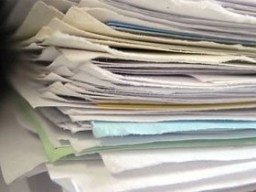We would be pleased if you find an error in the word problem, spelling mistakes, or inaccuracies and send it to us. Thank you!Tips to related online calculators
Do you want to convert length units?

## Next similar math problems:

• Wire cut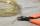A wire of length 7 m was cut into equal lengths using 4 cuts. How long is each piece?
• Chester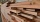Chester has a piece of wood measuring 1 2/3 cm he needs to cut it to pieces measuring 3/4 cm long. How many pieces of wood did Chester cut?
• PlumberPlumber had to cut the metal strip with dimensions 380 cm and 60 cm to the largest squares so that no waste. Calculate the length of the sides of a square. How many squares cut it?
• Paper squaresThe paper rectangle measuring 69 cm and 46 cm should be cut into as many squares as possible. Calculate the lengths of squares and their number.
• Dressmaker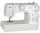Dressmaker left a piece of canvas shorter than 5 meters. She is deciding whether it sew a skirt or dress. The canvas was exactly as much as they consumed had cut up her skirt to 120 cm, or 180 centimeters dress. What a piece of canvas left her?
• Math test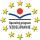In class 6.A are 26 students and the teacher had managed to give tests to 13 students. 13 testes he gave in 6.5 min. How many pupils still haven't test? How long will it take techer to give tests to 43 students?
• Wooden blockA cuboid-shaped wooden block has 6 cm length, 4 cm breadth, and 1 cm height. Two faces measuring 4 cm x 1 cm are colored in black. Two faces measuring 6 cm x 1 cm are colored in red. Two faces measuring 6 cm x 4 cm are colored in green. The block is divid
• RectanglesCalculate how many squares/rectangles of size 4×3 cm can be cut from a sheet of paper of 36 cm × 32 cm?
• AngieAngie poured 4 cartons of milk, each with 2.4 litres, into a mixing bowl. How many litres of milk were there altogether?
• Triangles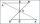Hanka cut the 20 cm long straws into three pieces each piece had a length in cm. Then, with these three pieces, she tried to make a triangle. a) What circuit has each of the triangles? b) How long can the longest side measure? c) How many different triang
• Apartment areaAnton wanted to measure the area of the apartment. But he bought a meter only 1.5m long. Later he recalled that he had two meters long at 4.5m and 18m at home. How many times are home gauges longer than the meter he bought?
• The cubeThe cube has an edge of 25 cm. We cut it into small cubes of 5 cm long side. How many of these little ones left when we build a new cube of 20 cm in length?
• Zhiwei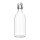Zhiwei wants to divide 19 litres of water equally into 6 pots. Find the volume of water in each pot?
• Wire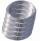From wire 53 meters long they cut of 12.1 m and then 13.1 m. How many meters of wire left?
• Cast brass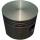Brass cast weight was 3.84 kg, it had turned into piston weight 3.491 kg. How many grams of brass was turned off?
• A section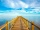A section of the boardwalk is made of 15 boards. Each board is 9 1/4 inches wide. The total width of the boardwalk is 144 inches. The spacing between each board is equal. What is the width of the spacing between the boards?
• SurianiSuriani had 3.05 m of ribbon. She used up 0.76 m of it. What is the length of ribbon that she has left?